### Home > CALC > Chapter 1 > Lesson 1.4.4 > Problem1-184

1-184.
1. As a cheetah runs, its velocity is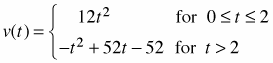where v(t) is measured in feet per second. Homework Help ✎

1. Sketch a graph of v(t) on your paper.

2. Approximately how fast is the cheetah running at t = 1 second? How did you get your answer?

3. To catch prey, such as an antelope, the cheetah runs for 3 seconds. Approximately how far does it need to run to catch its prey? Describe your method.

4. If the cheetah spots Annalou the Antelope standing 50 feet north of the Great Pond and runs north in a direct line towards her, catching her in exactly 3 seconds, what was the cheetah's initial position relative to the pond?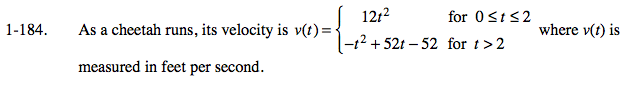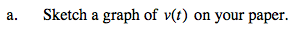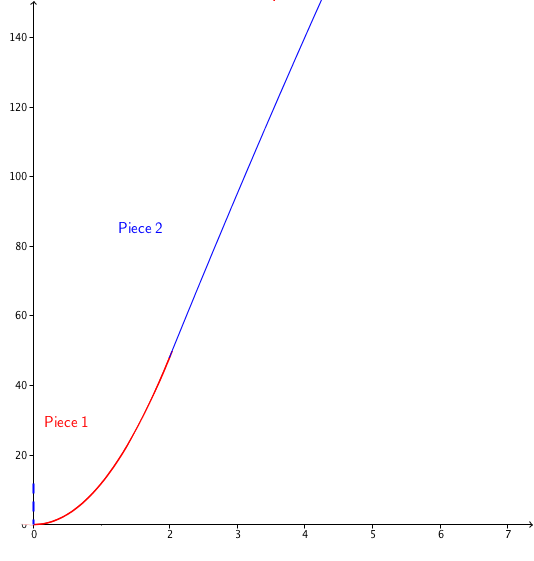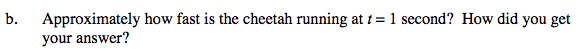This is a velocity function. So, what is the y value at x = 1?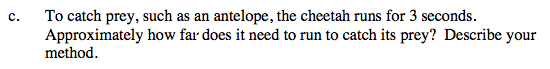Use geometry to approximate the area under the curve.

Trapezoid Method 107.5 ft
Midpoint Method 101.75 ft
There are other valid geometric methods.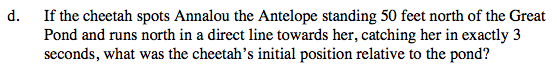Your answer in part (c) is an approximation of how far the cheetah traveled in 3 seconds. At t = 0, Annalou was only 50 feet north of the pond. Where was the cheetah?